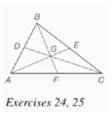Chapter 7.CR, Problem 24CRElementary Geometry For College St...

7th Edition
Alexander + 2 others
ISBN: 9781337614085

Solutions

Chapter
SectionElementary Geometry For College St...

7th Edition
Alexander + 2 others
ISBN: 9781337614085
Textbook Problem

Given: Δ A B C with medians A E , ¯ C D , ¯ B F ¯ Find: a) B G if B F = 18 b) G E if A G = 4 c) D G if C G = 4 3To determine

a)

To find: BG if BF=18.

Explanation

Given:

The triangle ABC with medians AE¯,CD¯,BF¯.

Theorem used:

The three medians of a triangle are concurrent at a point that is two-thirds the distance from any vertex to the midpoint of the opposite side.

Calculation:

We have BF=18

By the theorem, we get BF=32BG

To determine

b)

To find: GE if AG=4.

To determine

c)

To find: DG if CG=43.

Still sussing out bartleby?

Check out a sample textbook solution.

See a sample solution

The Solution to Your Study Problems

Bartleby provides explanations to thousands of textbook problems written by our experts, many with advanced degrees!

Get Started

In Exercises 1-6, simplify the expression. 2. 2a23ab9b22ab2+3b3

Applied Calculus for the Managerial, Life, and Social Sciences: A Brief Approach

Find the derivatives of the functions in Problems 1-34. 19.

Mathematical Applications for the Management, Life, and Social Sciences

Fill in each blank: 32floz=pt

Elementary Technical Mathematics

Find the limit. limx0sin5x3x

Single Variable Calculus: Early Transcendentals, Volume I

Convert the following percents to decimals. 76%

Contemporary Mathematics for Business & Consumers

If velocity is v(t) = 4t + 4 and s(1) = 2, then s(t) = 2t2 + 4t – 4 2t2 + 4t + 6 t2 + 4 t2 − 4

Study Guide for Stewart's Single Variable Calculus: Early Transcendentals, 8th

Define applied research and basic research and identify examples of each.

Research Methods for the Behavioral Sciences (MindTap Course List)